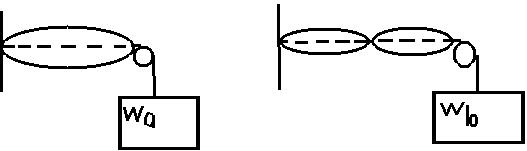Question# The drawing shows two strings that have the same lengthand the same linear density. The left end of each string is attached to a wall,while the right

Other
ANSWEREDThe drawing shows two strings that have the same lengthand the same linear density. The left end of each string is attached to a wall,while the right end passes over a pulley and is connected to objects of different weights(Wa and Wb). Different standing waves are set up on each string, but theirfrequencies are the same. If $$\displaystyle{W}_{{a}}={44}\ {N}$$, what is $$\displaystyle{W}_{{b}}$$.2021-03-23

The drawing shows two strings that have the samelength and the same lineardensity. The left end of each string is attached to a wall, while the right end passes over a pulley and isconnected to objects of different weights ($$\displaystyle{W}_{{a}}$$ and $$\displaystyle{W}_{{b}}$$). Different standing waves are set up on eachstring, but their frequenciesare the same.
If $$\displaystyle{W}_{{a}}={44}\ {N}$$ what is $$\displaystyle{W}_{{b}}$$ $$\displaystyle{\frac{{\lambda_{{a}}}}{{{2}}}}={l}$$
$$\lambda_a=2l$$
and $$\displaystyle\lambda_{{b}}=\lambda={l}$$
Since $$\displaystyle{V}=\lambda\cdot{v}=\sqrt{{{\frac{{{W}}}{{\mu}}}}}$$ or $$\displaystyle\lambda={\frac{{{1}}}{{{\left({v}\right)}\sqrt{{{\frac{{{W}}}{{\mu}}}}}}}}$$
For first: $$\displaystyle\lambda_{{a}}={\frac{{{1}}}{{{\left({v}\right)}\sqrt{{{\frac{{{W}_{{a}}}}{{\mu}}}}}}}}$$
For second: $$\displaystyle\lambda_{{b}}={\frac{{{1}}}{{{\left({v}\right)}\sqrt{{{W}_{{b}}}}{\left\lbrace\mu\right\rbrace}}}}$$
Note: Rest of the parts are constant
$$\displaystyle{W}_{{b}}={\frac{{{W}_{{a}}}}{{{4}}}}$$
$$\displaystyle={\frac{{{44}}}{{{4}}}}\ {N}$$
$$\displaystyle{W}_{{b}}={11}{N}$$